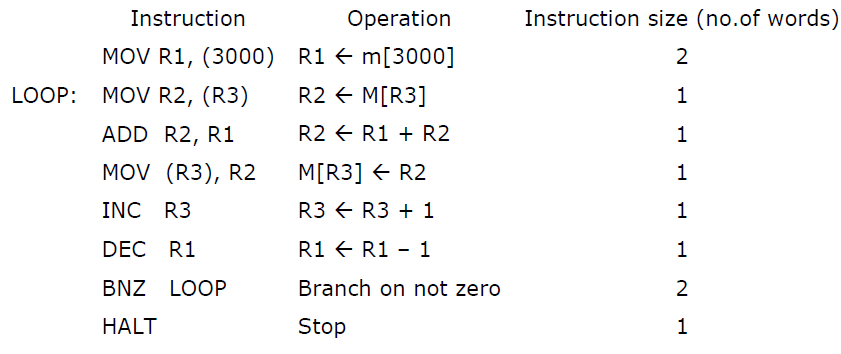# GATE | GATE-CS-2007 | Question 71

Consider the following program segment. Here R1, R2 and R3 are the general purpose registers.Assume that the content of memory location 3000 is 10 and the content of the register R3 is 2000. The content of each of the memory locations from 2000 to 2010 is 100. The program is loaded from the memory location 1000. All the numbers are in decimal. Assume that the memory is word addressable. The number of memory references for accessing the data in executing the program completely is:
(A) 10
(B) 11
(C) 20
(D) 21

Explanation: Explanation:

Ist memory reference R1←M and then in the loop which runs for 10 times, because the content of memory location 3000 is 10 given in question and loop will run 10 times as

R2← M[R3]

M[R3] ←R2

There are two memory reference every iteration

10*2=20

Total=20+1=21

So  (D) is correct option.

My Personal Notes arrow_drop_up
Article Tags :

Be the First to upvote.

Please write to us at contribute@geeksforgeeks.org to report any issue with the above content.